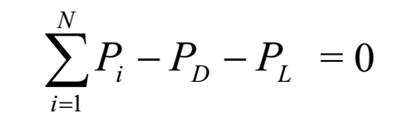• System constraints

Generally there are two types of constraints or restrictions, namely

1. Equality constraints
2. Inequality constraints

Equality constraints: The equality constraint represents the basic load flow equation involving active and reactive power.Inequality Constraints:

1. Generator Constraints:

PGmax limited by thermal considerations of boiler to keep the temperature rise within allowable limits.
PGmin limited by flame instability of boiler.
QGmax limited by stability limit of machine.
QGmin limited by overheating of rotor of generating unit.

2. Nodal Voltage Constraints:
The voltage magnitudes and phase angles at various nodes should lie within certain values.

VNmax & VNmin  limited to operate end users equipments satisfactorily.
δNmax limited due to transient stability.
δNmin limited for proper utilization of transmission line facility.

3. Transmission Line Constraints:
The flow of active power and reactive power in the transmission line is limited by the thermal capability of the circuit generally expressed as

SP ≤ CPmax

4. Transformer tap Constraints:
The range of a tap is limited by the physical construction of each transformer.

For an auto-transformer, the minimum tap settings is zero and maximum can be 1, i.e. 0 ≤ t ≤ 1.0

For two winding transformer, if there are tapping on the secondary side, we have 0 ≤ t ≤ n ; n is known as the transformation ratio.

For phase shifting transformer, phase shift limit should be θmin ≤ θ ≤ θmax.

5. Running Spare Capacity Constraints:
These constraints are essential when we have to meet

a) A forced outage of one or more alternators within the system

b) An unexpected load which may be applied on the system.

The total power generation should be such that it should meet load demand, various losses and minimum spare capacity, i.e. PG ≥ PD+ PL+PSC.

Here PG is the total generation, PD is the total power demand, PL is the total power loss and PSC is some pre-specified spare capacity. A well planned system must have a very less value of PSC.

6. Network Security Constraints:
If, initially the system is operating satisfactorily and thereafter there is an outage, it could be scheduled or forced one; then it is natural that some of the constraints of the system may be violated.

The complexity of those constraints (in term of number of constraints) is increased when a large system is being considered. For this, a study is made with outage of one branch at a time and then more than one branches at a time. The nature of these constraints are same as voltage and transmission line constraints.